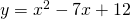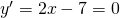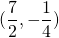# Solution assignment 01 Optimizing f(x)

### Assignment 1

Determine the extreme of the parabola:### Solution

We know that the graph of this function is an 'opens up' parabola and thus it has a minimum.
We differentiate the function and solve the equation:The solution of this equation is:and the coordinates of the minimum are:0